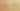VariablesHoldObjects

Misconception:

Any variable, such as a in a = 1, directly holds the value of the object.Incorrect

A variable contains a whole object

Correct

A variable contains a reference to an object

CorrectionHere is what's right.

All variables in Python store references to objects.

A useful built-in function id(obj) returns the “identity” of obj. You can think of “identity” as the object’s memory address. In CPython, the reference implementation of the Python programming language, id(x) indeed returns the memory address where x is stored.

As an example, consider the output of the following code:

x = 1
print(x)      # displays the value of the object x refers to
print(id(x))  # displays the reference stored in x
x = 2
print(id(x))

SymptomsHow do you know your students might have this misconception?

A common symptom of this misconception is the special case where students draw diagrams of memory and some variable boxes contain the values instead of references to them.

ValueHow can you build on this misconception?

This is a great opportunity to start discussing the idea of aliasing: two variables that refer to the same object. One could not create aliases if objects had to live inside variables.

Python